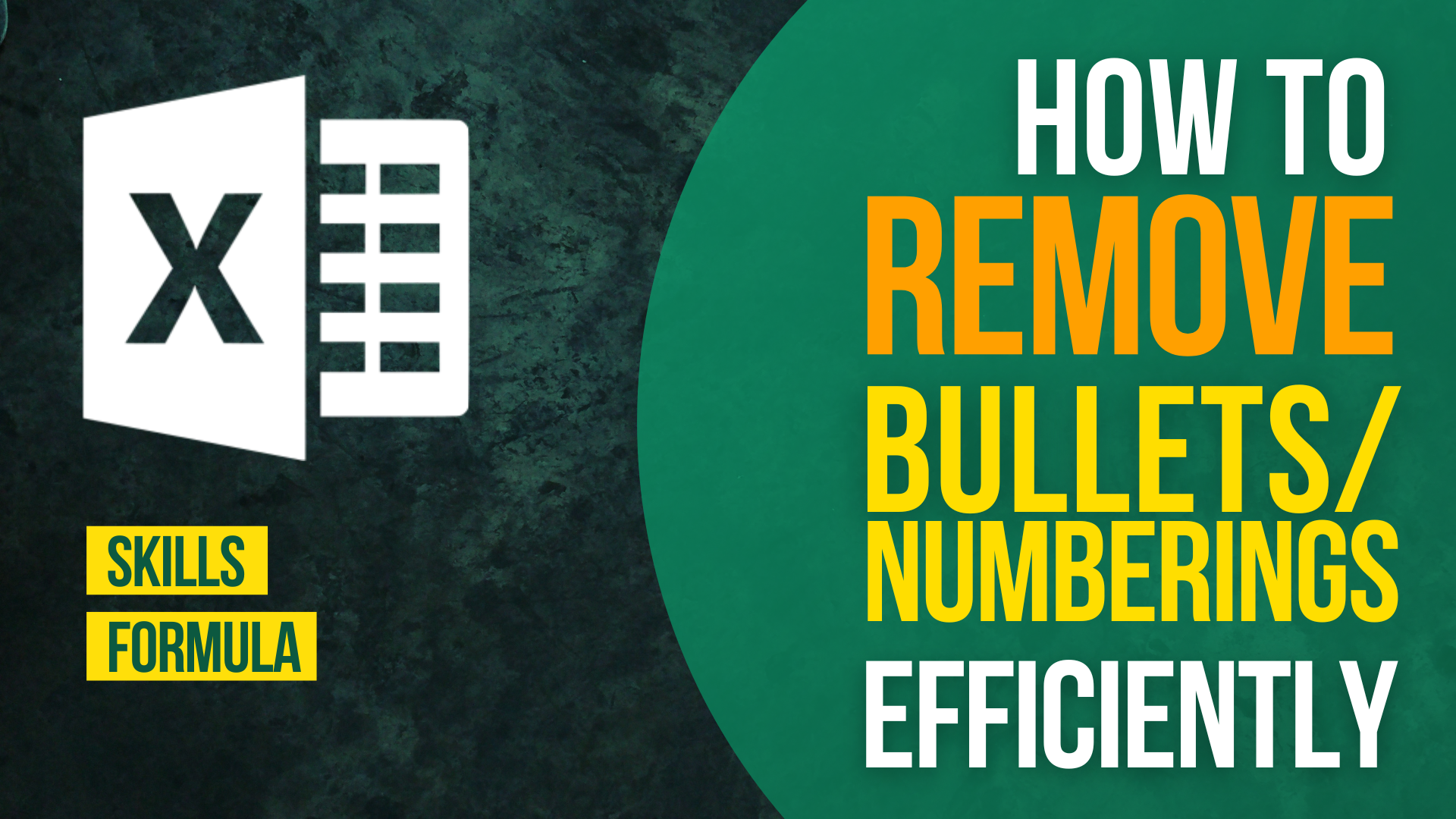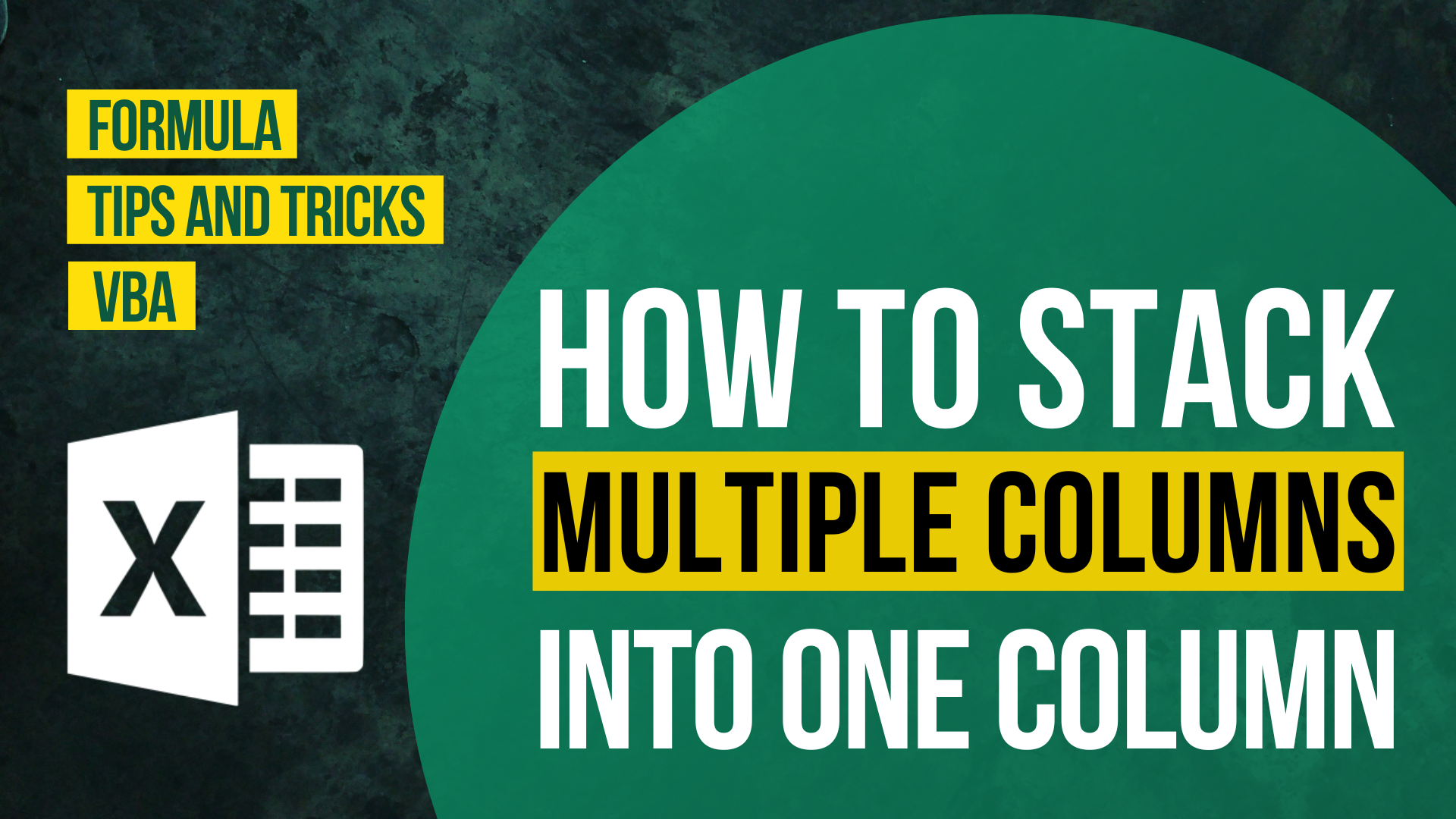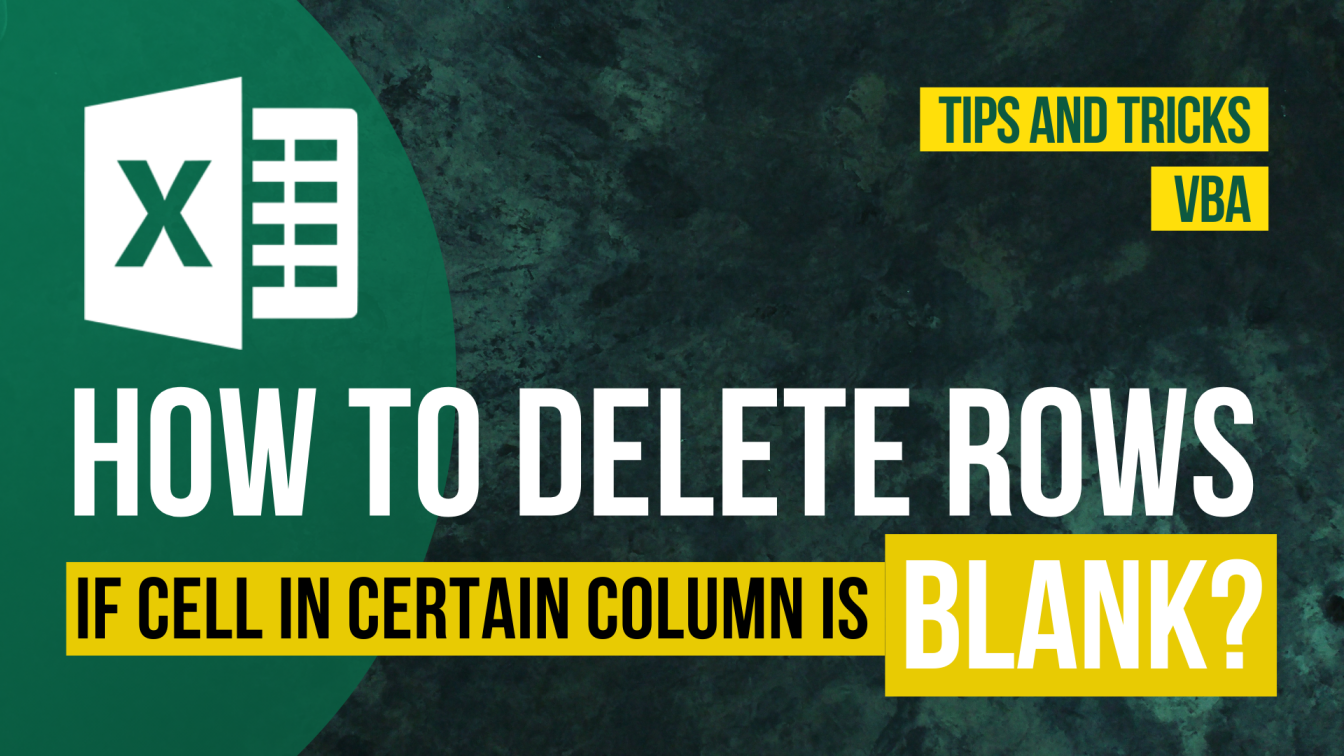# How to Unpivot or Reverse Pivot in ExcelThere are numerous ways of presenting data in Excel. One of the most popular way is to present data in crosstab format. To turn tabular data into crosstab format (which is pivoting) is not so difficult. We can do that by using an in-built function called Pivot table. However, to unpivot data is not as easy as to pivot it.

In this article, I will show you how to unpivot or reverse pivot data by VBA. I will guide you through how to use the VBA script and provide you with different scripts to cater for your own situation.

**Read How to Insert & Run VBA code in Excel – VBA101 if you forget how to insert VBA code in Excel.

If you have the following questions, I suggest you reading this until the end.

• How to unpivot data in excel?
• How to Unpivot or Reverse Pivot in Excel?
• How to turn crosstab format data into tabular format in Excel?

You might also be interested in How To Change The Default Number Of Sheets In Excel

## Example

Here we have two tables.

The table on the left is the original table. As we can see, the table is in crosstab format. There are both column header and row header.

The table on the right is the unpivoted table. It is in tabular format. There isn’t any header.

### What we would like to do

`To unpivot the data`

You might also be interested in Edit The Same Cell In Multiple Excel Sheets

## Unpivot or Reverse Pivot by VBA

Read How to Insert & Run VBA code in Excel – VBA101 if you forget how to insert VBA code in Excel.

When the VBA script start to run, there will be 2 pop up windows showing.

When you see a pop-up window asking for a range, select the range of the cells without the row header or column header.

In the above example, I selected cell B2 to cell F4.

When you see a pop-up window asking for a range to place the end result, select any range you like.

```Sub Unpivot_rowheaderfirst()
'Dim Rng As Range
Dim PivotRng As Range
Dim aCell As Range

'Use inputbox to collect a range of aCells to be pivoted
Set Rng = Application.InputBox("Select a range of cells to be pivoted", "Range selection", , , , , , 8)
'Use inputbox to a cell to place the pivoted data
Set PivotRng = Application.InputBox("Select a cell to place the pivoted data", "Final Destination", , , , , , 8)

For Each aCell In Rng
If aCell.Value <> "" Then
PivotRng.Activate
PivotRng.Value = aCell.Offset(0, -(aCell.Column - Rng.Column + 1)).Text
PivotRng.Offset(0, 1).Value = aCell.Offset(-(aCell.Row - Rng.Row + 1), 0).Text
' get the value
PivotRng.Offset(0, 2).Value = aCell.Text
' move cell to next row
Set PivotRng = PivotRng.Offset(1, 0)
End If
Next

End Sub```

```Sub Unpivot_columnheaderfirst()
'Dim Rng As Range
Dim PivotRng As Range
Dim aCell As Range

'Use inputbox to collect a range of aCells to be pivoted
Set Rng = Application.InputBox("Select a range of cells to be pivoted", "Range selection", , , , , , 8)
'Use inputbox to a cell to place the pivoted data
Set PivotRng = Application.InputBox("Select a cell to place the pivoted data", "Final Destination", , , , , , 8)

For Each aCell In Rng
If aCell.Value <> "" Then
PivotRng.Activate
PivotRng.Value = aCell.Offset(-(aCell.Row - Rng.Row + 1), 0).Text
PivotRng.Offset(0, 1).Value = aCell.Offset(0, -(aCell.Column - Rng.Column + 1)).Text
' get the value
PivotRng.Offset(0, 2).Value = aCell.Text
' move cell to next row
Set PivotRng = PivotRng.Offset(1, 0)
End If
Next

End Sub

```

Hungry for more useful Excel tips like this? Subscribe to our newsletter to make sure you won’t miss out on any of our posts and get exclusive Excel tips!

### Other VBA articles

How to Check/Test if Sheets Exist in Excel?

How To List All Worksheets Name In A WorkbookHow to Input Time Duration in ExcelHow to Remove Bullets/Numberings in Excel

Popular posts